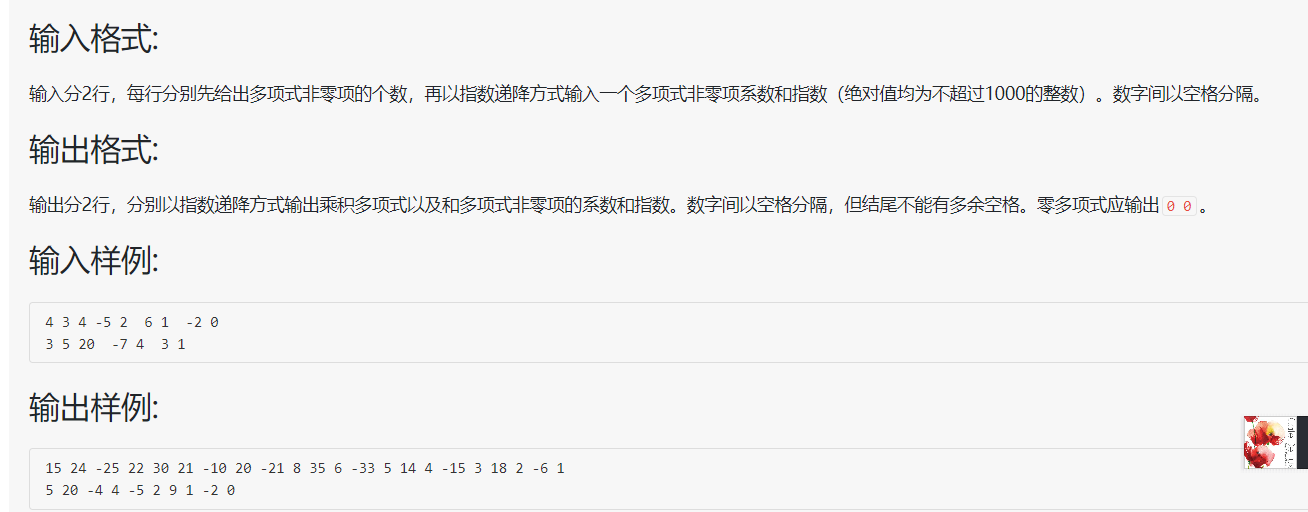2020-02-25 09:43

# c语言,一元多项式相乘,相乘结果莫名少第一项4 3 4 -5 2 6 1 -2 0

3 5 20 -7 4 3 1

``````#include<stdio.h>
#include<stdlib.h>
typedef struct Node *Poly;
struct Node{
int coef;
int expon;
};
void Attach(int c,int e,Poly *R);
Poly Sum(Poly p1,Poly p2);
Poly Mult(Poly p1,Poly p2);
void print(Poly p);
void freep(Poly p);
int main(void)
{
Poly p1,p2;
print(Mult(p1,p2));
print(Sum(p1,p2));
return 0;
}
{
int N,c,e;
Poly p,Rear,t;
scanf("%d",&N);
p = (Poly)malloc(sizeof(struct Node));
Rear = p;
while(N--)
{
scanf("%d %d",&c,&e);
Attach(c,e,&Rear);
}
t = p;
free(t);
return p;
}
void Attach(int c,int e,Poly *R)
{
Poly p;
p = (Poly)malloc(sizeof(struct Node));
p->coef = c;
p->expon = e;
*R = p;
}
Poly Sum(Poly p1,Poly p2)
{
t1 = p1;
t2 = p2;
while(t1&&t2)
{
if(t1->expon == t2->expon)
{
if(t1->coef + t2->coef)
{
Attach(t1->coef+t2->coef,t1->expon,&Rear);
}
}
else if(t1->expon > t2->expon)
{
Attach(t1->coef,t1->expon,&Rear);
}
else
{
Attach(t2->coef,t2->expon,&Rear);
}

}
while(t1)
{
Attach(t1->coef,t1->expon,&Rear);
}
while(t2)
{
Attach(t2->coef,t2->expon,&Rear);
}
free(t);
}
Poly Mult(Poly p1,Poly p2)
{
int c,e;
if(!p1||!p2)
{
return NULL;
}
t1 = p1;
t2 = p1;
while(t2)
{
Attach(t1->coef*t2->coef,t1->expon+t2->expon,&Rear);
}
while(t1)
{
t2 = p2;
while(t2)
{
c=t1->coef*t2->coef;
e=t1->expon+t2->expon;
{
}
{
{
}
else
{
free(t);
}
}
else
{
Attach(c,e,&Rear);
}
}
}
free(t);
}
void print(Poly p)
{
int flag = 0;
if(!p)
{
printf("0 0\n");
return ;
}
while(p)
{
if(!flag)
{
flag = 1;
}
else
{
printf(" ");
}
printf("%d %d",p->coef,p->expon);
}
printf("\n");
}

``````
• 点赞
• 写回答
• 关注问题
• 收藏
• 邀请回答